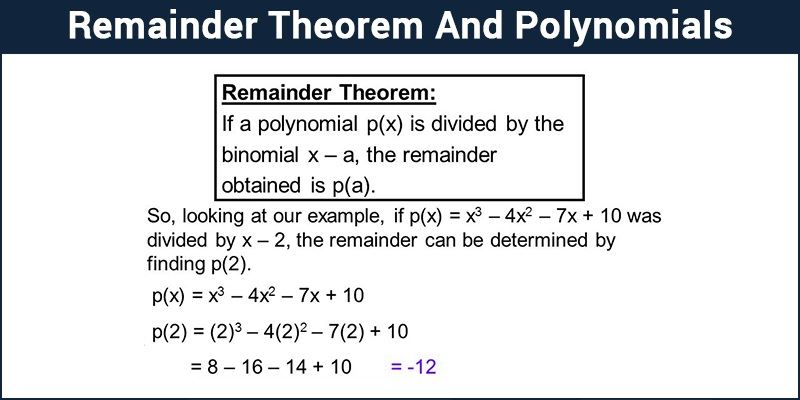Jet Set Go! All about Aeroplanes Jet Set Go! All about Aeroplanes

# Remainder Theorem And Polynomials

An expression of the form axn + bxn-1 + cxn-2 + ………………. + kx + l, where each variable has a constant accompanying it as its coefficient, is called a polynomial of degree ‘n’ in variable x. Thus, a polynomial is an expression in which a combination of a constant and a variable is separated by an addition or a subtraction sign. Each variable separated with an addition or subtraction symbol in the expression is known as a term. The maximum power of the variable in a polynomial expression is the degree of the polynomial. Let’s learn about the remainder theorem of polynomials.## Remainder Theorem

When we divide a number, for example, 25 by 5 we get 5 as quotient and 0 as the remainder. This can be expressed as:

Dividend = (Divisor × Quotient) + Remainder

i.e, 25= (5 x 5) + 0

Here the remainder is zero thus we can say 5 is a factor of 25 or 25 is a multiple of 5. Thus reminder gives us a link between dividend and the divisor. We can divide a polynomial by another polynomial and can express in the same way.

Let’s divide a polynomial, p(x) = 4x2 + x – 1 by another polynomial (x + 1). After a long division we will get quotient, q(x) = 4x-3 and remainder, r(x) = 2. This can be expressed as:

4x2 + x – 1=(x + 1) × (4x-3) + 2

Suppose p(x) and g(x) are two polynomials where the degree of p(x) > g(x) and g(x) ≠0. When we divide p(x) by g(x), if we get the polynomial q(x) as quotient and r(x) as remainder, then this can be expressed as:

p(x) = g(x) × q(x) + r(x)

The remainder theorem of polynomials gives us a link between the remainder and its dividend. Let p(x) be any polynomial of degree greater than or equal to one and ‘a’ be any real number. If p(x) is divided by the linear polynomial x – a, then the remainder is p (a). This is the remainder theorem. It helps us to find the remainder without actual division. Let’s take a look at the application of the remainder theorem with the help of an example.

Example 1: Find the remainder when t3 – 2t2 + t + 1 is divided by t – 1.

Solution: Here, p(x) = t3 – 2t2 + t + 1, and the zero of t – 1 is 1.

∴ p (1) = (1)3 – 2(1)2 + 1 + 1= 1

By the Remainder Theorem, 1 is the remainder when t3 – 2t2 + t + 1 is divided by t – 1.